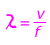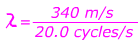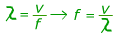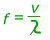# Wavelength Formula

Wavelength Formula

Wavelength is the distance between the crests of a wave. Many different things can move like waves, like strings, water, the air (sound waves), the ground (earthquakes), and light can be treated as a wave. Wavelength is represented with the Greek letter lambda: λ. It is equal to the velocity of the wave, divided by the frequency. Wavelength is expressed in units of meters (m).λ = wavelength, the distance between wave crests (m)

v = wave velocity, the speed that waves are moving in a direction (m/s)

f = frequency, the wave crests that go through a point in a certain time (cycles/s or Hz)

Wavelength Formula Questions:

1) The speed of sound is about 340 m/s. Find the wavelength of a sound wave that has a frequency of 20.0 cycles per second (the low end of human hearing).

Answer: The wave velocity v = 340 m/s, and the frequency f = 20.0 cycles/s. The wavelength ? can be found using the equation:λ = 17.0 m

The wavelength of the sound is 17 meters.

2) A motor boat makes waves that travel across the surface of a lake. The waves travel toward shore at a velocity of 1.50 m/s. The distance between wave crests is 2.00 m. What is the frequency of the waves?

Answer:: The wave velocity v = 1.50 m/s, and the wavelength λ = 2.00 m. The frequency must be solved for, so rearrange the equation:f = 0.75 waves/s

The frequency of these water waves is 0.75 waves per second.